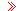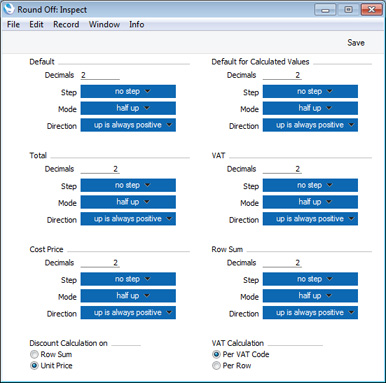Language:

Version 8.4hansaworld.comFAQForums

# HansaManualsIntroduction to Settings in the System ModuleAccess GroupsActive UsersAuto ActionsBank HolidaysBase CurrencyBase Currency RatesCompany Date and Numeric FormatCompany InfoConfigurationConversions - Master, Conversions - Accounts and VAT CodeConversions - Master, Conversions - Activity TypesConversions - Master, Conversions - Contact ClassificationsConversions - Master, Conversions - ContactsConversions - Master, Conversions - Currency CodesConversions - Master, Conversions - ItemsConversions - Master, Conversions - LocationsConversions - MasterConversions - Master, Conversions - SuppliersConversions - Master, Conversions - Tags/ObjectsConversions - Master, Conversions - UsersCountriesCountries RegionsCurrency Round OffDays and MonthsDepartmentsDiscount OptionsDisplay GroupsExchange Rates SettingsForm SettingsGlobal Warnings on UnOKed RecordsInternet EnablersJournalingKPIsLanguagesLockingLocking ExceptionsNature of BusinessNumber Series DefaultsOpened Windows HistoryOptional FeaturesPassword SecurityPrintersReporting PeriodsRound OffSales GroupsSingle FunctionsSpecial DaysStylesSub-ledger Control AccountsTask Manager AccessUser Warnings on UnOKed RecordsValues in TextVAT Reg. Number MasksWeeksTutorials for Standard Accounts

Round Off

This page describes the Round Off setting in the System module.

---

Use the Round Off setting to control how different figures will be rounded.

Rounding throughout the system. When you enter a Sub System record (i.e. a record that will eventually post to the Nominal Ledger, such as an Invoice or Purchase Invoice), several figures in that record will be subject to the rounding process. When you then mark the record as OK and save, causing a Nominal Ledger Transaction to be created automatically, any amounts lost or gained due to the rounding process will be posted to the Round Off Gain or Round Off Loss Accounts specified in the Account Usage S/L setting. These Accounts will be used in Transactions generated from all Sub System records (e.g. in Transactions generated from both Sales and Purchase Invoices).

There are two settings that control rounding: this one, and the Currency Round Off setting, also in the System module. When you enter a Sub System record, rounding rules will be taken from the row in the Currency Round Off setting for the Currency/Payment Term/Register combination. If there is no row in the Currency Round Off setting for that combination, rounding rules will be taken from this setting. The Currency Round Off setting only affects rounding for VAT, row sums and totals.

To open the Round Off setting, ensure you are in the System module using the [Module] button in the Master Control panel and click the [Settings] button, also in the Master Control panel. The 'Settings' list opens: double-click 'Round Off'. When the 'Round Off: Inspect' window opens, enter your requirements for the rounding process as necessary. Click [Save] to save and close, or the close box to close without saving changes.The window contains six sections where you can specify how particular figures will be rounded (Default, Default for Calculated Values, Total, VAT, Cost Price and Row Sum). Each section contains the same set of options that control how the rounding process will operate. First we will describe these options (the Decimals field, and the Step, Mode and Direction pop-up menus), then we will describe the sections of the window.
Decimals
Specify the number of decimal places that you need. Example values are:
2
Rounds to two decimal places.

1
Rounds to one decimal place.

0
Rounds to the nearest whole number.

-1
Rounds to the nearest 10.

-2
Rounds to the nearest 100.
The maximum number of decimal places is seven. However, some non-calculated fields can only contain a maximum of three decimal places. For example, the Quantity and Unit Price fields in an Invoice can only contain up to three decimal places, and therefore the maximum number of decimal places in the calculated Sum field is six.

Step
Choose an option from the Step pop-up to specify the rounding increment, as follows:
no step
A rounding increment of 1 will be used. For example, if you are rounding to two decimal places, values after rounding will follow the sequence 0.00, 0.01, 0.02, 0.03 and so on. 0.023 will be rounded to 0.02 using this option.

5
A rounding increment of 5 will be used. For example, if you are rounding to two decimal places, values after rounding will follow the sequence 0.00, 0.05, 0.10, 0.15 and so on. 0.023 will be rounded to 0.00 using this option.

2.5
A rounding increment of 2.5 will be used. For example, if you are rounding to two decimal places, values after rounding will follow the sequence 0.00, 0.025, 0.05, 0.075 and so on. 0.023 will be rounded to 0.025 using this option. Note that this option will sometimes result in one extra decimal place than specified being used.
Mode
Choose an option from the Mode pop-up to specify how 0.5 will be rounded. The options are:
half up
0.5 will be rounded up to 1.0 when rounding to zero decimal places.

half down
0.5 will be rounded down to 0.0 when rounding to zero decimal places.

to even
0.5 will be rounded to the nearest even whole number. For example, when rounding to zero decimal places, 1.5 will be rounded to 2, 2.5 will be rounded to 2, 3.5 will be rounded to 4.

to odd
0.5 will be rounded to the nearest odd whole number. For example, when rounding to zero decimal places, 1.5 will be rounded to 1, 2.5 will be rounded to 3, 3.5 will be rounded to 3.

truncate
Rounding will be carried out by removing decimals. For example, 1.999 will be rounded to 1.99 (when rounding to two decimal places), to 1.9 (one decimal place) or to 1 (zero decimal places).
Direction
The Direction will only be used if the Mode is "half up" or "half down". It specifies what "up" and "down" mean for negative numbers.
up is always positive
If the Mode is “half up”,-1.5 will be rounded up to -1, +1.5 will be rounded up to 2.

If the Mode is “half down”, -1.5 will be rounded down to -2, +1.5 will be rounded down to 1.

up is away from zero
If the Mode is “half up”, -1.5 will be rounded up to -2, +1.5 will be rounded up to 2.

If the Mode is “half down”, -1.5 will be rounded down to -1, +1.5 will be rounded down to 1.

This option will ensure a negative record will completely cancel a positive one.
The 'Round Off: Inspect' window contains the following sections:
Default
The Default section has the following effects:
• It controls the number of decimals that will be displayed in Price fields and in fields with calculated values (e.g. Sum). For example, if the Default number of decimals is 4, four decimals will always be displayed. So, a value of 1 will be displayed as 1.0000, and 1.25 will be displayed as 1.2500.

• It sets the maximum number of decimals that can be used anywhere in this setting (except the Cost Price section). For example, if the Default number of decimals is 4, the Row Sum will be rounded to four decimal places, even if you specified in the relevant section of this setting that it should be rounded to six.

• The Quantity field in all matrices will not follow the number of decimal places specified here. This field can support up to three decimal places. For example, if you enter "1", "1" will be displayed in the field, if you enter "1.123", "1.123" will be displayed in the field, and so on. If you enter "1.1234", then it will be rounded to three decimal places following the Default rules for Step, Mode and Direction.

• The Unit Price field in all matrices can also support up to three decimal places, and will always display a minimum of two (even if the Default number of decimals is less than two). For example, if you enter "1", "1.00" will be displayed in the field, if you enter "1.123", "1.123" will be displayed in the field, and so on. If you enter "1.1234", then it will be rounded to three decimal places following the Default rules for Step, Mode and Direction. If the Default number of decimals is greater than three, then the appropriate number of trailing zeros will be added to the figures. For example, if the Default number of decimals is five and you enter "1.12", "1.12000" will be displayed.
Default for Calculated Values
This section controls the rounding of calculated figures not mentioned elsewhere in this setting (for example, figures resulting from Currency conversions). The number of decimals should not be more than the Default number of decimals specified above.

Total
This section controls the rounding of the figures shown in the TOTAL field below all matrices. The number of decimals should not be more than the Default number of decimals specified above.

VAT
This section controls the rounding of the VAT calculation in each matrix row (but the figures shown in the VAT field below all matrices are rounded using the Calculated Values section above). The number of decimals should not be more than the Default number of decimals specified above.

Calc Cost Value
This section allows you to determine the rounding method to be used when stock values are calculated.

Whenever the Weighted Average or Queued value of an Item is updated (e.g. when you mark a Goods Receipt as OK and save it), the calculation will use the rounding method specified here. Weighted Average or Queued values will then be shown in the Stock List report and will be used to calculate the values of outgoing stock transactions.

If you have chosen to have the Cost Prices of your Items updated automatically from Goods Receipts, the updating of Item Cost Prices will also use this rounding method.

The number of decimals that you specify here can be more than the Default number of decimals specified above.

The Currency Round Off setting does not contain rounding options for Cost Prices. Therefore, rounding rules for Cost Prices will always be taken from this Round Off setting.

Row Sum
These options control the rounding used in the calculation of the figures shown in the Sum field (Qty * Unit Price) in all matrices and consequently in the Subtotal field below matrices as well. The number of decimals should not be more than the Default number of decimals specified above.

Discount Calculation
This group of options allows you to determine how discounts are calculated in sales transactions (i.e. in Quotations, Sales and Purchase Orders, Invoices, Contracts, Work Sheets and Project Budgets). In certain circumstances (where there is a very small unit price and a large quantity), the two alternatives can produce different results due to rounding. If you choose the Row Sum option, the discount will be calculated after the multiplication of the unit price and the quantity. If you choose the Unit Price option, the discount will be applied to the unit price before it is multiplied by the quantity.

For example, you receive an Order for 20,000 items priced at 0.05 with a 25% discount. Using the Row Sum option, the calculated discount is 250:
20000 x 0.05 = 1000. 25% of 1000 = 250
Using the Unit Price option, the calculated discount is 200:
25% of 0.05 rounded to two decimal places is 0.01. 0.01 x 20000 is 200
VAT Calculation
Specify here how VAT is to be rounded off.
Per VAT Code
VAT subtotals will be calculated for each VAT Code used in a transaction. These subtotals will then be rounded as determined in the Currency Round Off setting or using the option chosen above (whichever is appropriate). The subtotals will then be added together to produce the overall VAT total.

Per Row
The VAT amount for each individual transaction row will be rounded as determined in the Currency Round Off setting or using the option chosen above (whichever is appropriate). These amounts will then be added together to produce the overall VAT total.
Note that the transaction row sum will be rounded as determined in the Currency Round Off setting or using the option chosen above (whichever is appropriate) before the VAT calculation is carried out.
---

Settings in the System module:

Go back to: